Maths-
General
Easy

Question

# If a hyperbola passing through the origin has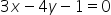and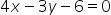as its asymptotes, then the equation of its tranvsverse and conjugate axes are

##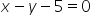and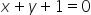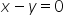and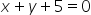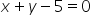and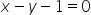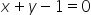andHint:

## The correct answer is:and### a line or curve that serves as the boundary of another line or curve in mathematics. An example of an asymptotic curve is a descending curve that approaches but does not reach the horizontal axis, which is the asymptote of the curve.The transverse axis is the bisector containing origin, and the hyperbola's axes are the bisectors of the pair of asmptodes.So we have:Here we used the concept of polar coordinate system and also the trigonometric ratios to find the solution.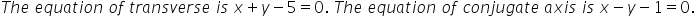#### With Turito Foundation.#### Get an Expert Advice From Turito.